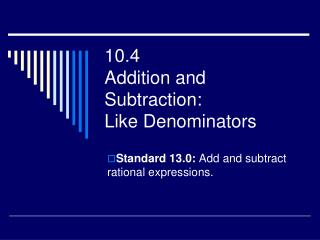DownloadDownload Presentation10.4 Addition and Subtraction: Like Denominators

# 10.4 Addition and Subtraction: Like Denominators

Télécharger la présentation## 10.4 Addition and Subtraction: Like Denominators

- - - - - - - - - - - - - - - - - - - - - - - - - - - E N D - - - - - - - - - - - - - - - - - - - - - - - - - - -
##### Presentation Transcript

1. 10.4Addition and Subtraction:Like Denominators Standard 13.0: Add and subtract rational expressions.

2. Adding and Subtracting Fractions: Like Denominators

3. Adding and Subtracting Rules: Like Denominators • Change Subtraction to Add the Opposite • Factor the Denominator • Add the Numerator • Factor the Numerator if possible • Simplify if possible

4. #3 Add or subtract. Simplify Add the numerator and keep the denominator

5. #4 Add or subtract. Simplify Add the numerator and keep the denominator

6. #5 Add or subtract. Simplify

7. #6 Add or subtract. Simplify -28 -7 4 -3